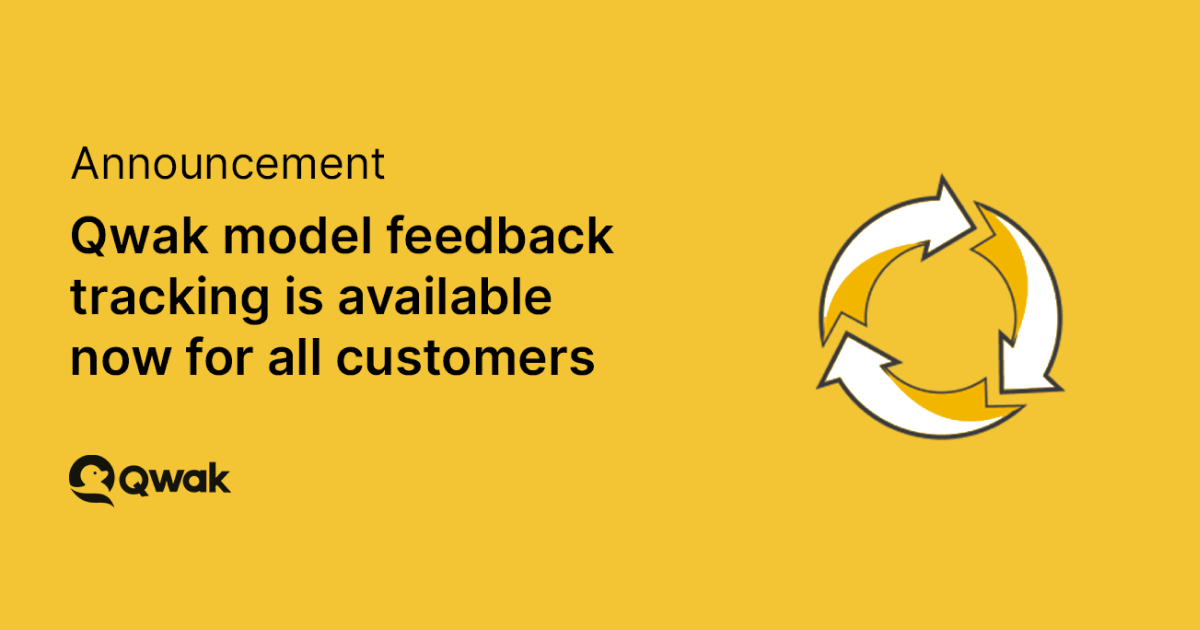x
Back to blogQwak now supports machine learning (ML) models feedback tracking.

ML models are trained on data, and understanding the difference between the model trained data and current consumed data is a complicated task.  This new capability allows data scientists to monitor their actual model performance in real time and connect the deployed, invoked model to its real metrics.

There are some advantages of using feedback tracking for ML Models.

• Actual metrics — Tracking the feedback loop allows data scientists to understand the model's actual performance and not just the model training performance.
• Retraining based on performance — When data scientists understand the model performance, they can make a data-based decision regarding model retraining.
• Labeled data set — The feedback data connected with the model analytics data is a labeled dataset that can be used for future training.

## How it works

Once a model is deployed to Qwak using one of the deployment options, with Qwak Analytics enabled, all the input data and outputs of the model are automatically tracked.. The feedback API is used to send tagged labels and connect them to the same model ID. Qwak feedback joins the two data sources and calculates the model metrics.

## Getting started with model feedback tracking

To collect the model inference data, the Analytics feature should be enabled. But having the data saved in the Qwak lake isn’t enough; in order to close the feedback loop, an entity ID should be used. That entity ID will be the connection between an inference and a feedback data.

For instance, in our churn example, that can be created using the following command:

``````
@qwak.analytics()
def predict(self, df):
df = df.drop(['User_Id'], axis=1)
return pd.DataFrame(self.catboost.predict_proba(df)[:, 1],
columns=['Churn_Probability'])
``````

The predict function gets a dataframe that includes the entity ID, —in the churn model case, user_id — and drops it because it’s not needed for inference. The user_id will be logged in the Qwak lake, and it can be used for feedback.

Once data is saved in analytics, feedback calls can be sent as well.

``````
from qwak.inference.clients import FeedbackClient
feedback_client = FeedbackClient(model_id='churn_model')
feedback_client.actual(entity_name='User_Id', entity_value='fc9df0df7a5b',
tag = 'Churned', actuals=['1'])
``````

The feedback calls are connected to a specific model and use the same entity_id that was saved by the model. Different tags can be used for different use cases. In our case, each time a user has churned, we’ll send one to the feedback API.

In the UI, an automatic visualization will be created:

A few options can be configured through the UI:

1. The prediction timeline that would be used for feedback calculation.
2. What the system should do with predictions without feedback (in our case, calculate them as not churned).
3. The cutoff ratio that will be used to calculate whether a prediction is True or False.

Using this simple visualization, a model performance can be calculated and used to validate whether a model is still relevant or not.